# In each of the figures [(i)-(iv)] given below,

Question:

In each of the figures [(i)-(iv)] given below, a line segment is drawn parallel to one side of the triangle and the lengths of certain line-segment are marked. Find the value of x in each of the following :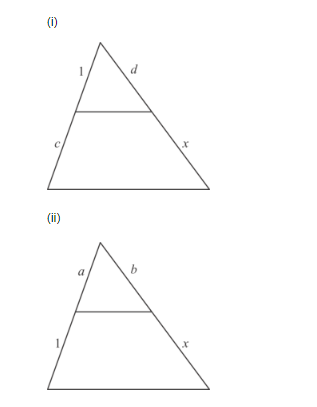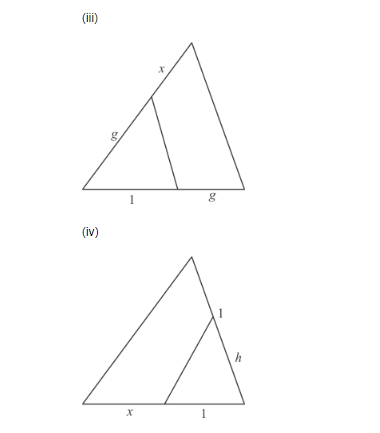Solution:

In each of the figure, we have to find the value of x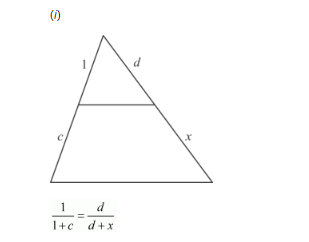By cross multiplication on both sides, we get

$1 \times(d+x)=d \times(1+c)$

$d+x=d+d c$

$x=d+d c-d$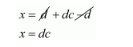Hence the value of $x$ is $d c$.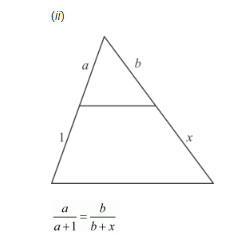By cross multiplication on both sides, we get

$a \times(b+x)=b \times(a+1)$

$a b+a x=a b+b$

$a x=a b+h-a b$

$a x=a b+b-a b$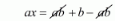$a x=b$

$x=\frac{b}{a}$

Hence the value of $x$ is $\frac{b}{a}$.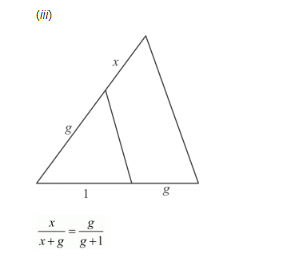By cross multiplication on both sides, we get

$x \times(g+1)=g \times(x+g)$

$x g+x=g x+g^{2}$

$x=g x+g^{2}-g x$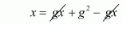$x=g^{2}$

Hence the value of $x$ is $g^{2}$.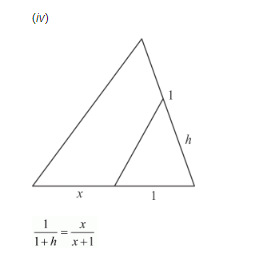By cross multiplication on both sides, we get

$1 \times(x+1)=x \times(1+h)$

$x+1=x+h x$

$x+1-x=h x$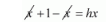$1=x h$

$\frac{1}{h}=x$

Hence the value of $x$ is $\frac{1}{h}$.